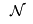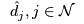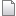# The Transshipment Problem in AMPL

The formulation of the transshipment problem in AMPL we present here is a straightforward translation of the alternative mathematical programme for the transshipment problem. We will build the file transshipment.mod.

The setis declared as NODES:

set NODES;


The net demandis declared as an integer parameter (note there is no >= 0):

param NetDemand {NODES} integer;


Note that the set NODES and the parameter NetDemand can be easily created from SUPPLY_NODES, DEMAND_NODES, Supply, etc, e.g.,:

set SUPPLY_NODES;
set DEMAND_NODES;
set TRANSSHIPMENT_NODES;

set NODES := SUPPLY_NODES union
TRANSSHIPMENT_NODES union
DEMAND_NODES;

param Supply {SUPPLY_NODES} >= 0, integer;
param Demand {DEMAND_NODES} >= 0, integer;

param NetDemand {n in NODES} integer
:= if n in SUPPLY_NODES then -Supply[n]
else if n in DEMAND_NODES then Demand[n]; # else 0 by default


The set ARCS is defined between pairs of nodes and costs and bounds are also defined:

set ARCS within NODES cross NODES;

param Cost {ARCS};

param Lower {ARCS} >= 0, integer, default 0;
param Upper {(i, j) in ARCS} >= Lower[i, j], integer, default Infinity;


Now, the mathematical programme follows directly:

var Flow {(i, j) in ARCS} >= Lower[i, j], <= Upper[i, j], integer;

minimize TotalCost:
sum {(i, j) in ARCS} Cost[i, j] * Flow[i, j];

subject to ConserveFlow {j in NODES}:
sum {(i, j) in ARCS} Flow[i, j] - sum {(j, k) in ARCS} Flow[j, k] >= NetDEmand[j];


-- TWikiAdminGroup - 22 Apr 2008

Topic attachments
I Attachment History Action Size Date Who Commentmod transshipment.mod r2 r1 manage 1.5 K 2008-04-28 - 02:12 MichaelOSullivan

This topic: OpsRes > WebHome > NetworkOptimisation > TransshipmentProblem > TransshipmentProblemInAMPL
Topic revision: r4 - 2008-04-28 - MichaelOSullivan

Copyright © 2008-2022 by the contributing authors. All material on this collaboration platform is the property of the contributing authors.
Ideas, requests, problems regarding TWiki? Send feedback